# Expressions

Let k represent an unknown number, express the following expressions:

1. The sum of the number n and two
2. The quotient of the number n and nine
3. Twice the number n
4. The difference between nine and the number n
5. Nine less than the number n

Result

k1 = (Correct answer is: n+2)k2 = (Correct answer is: n/9)k3 = (Correct answer is: 2n)k4 = (Correct answer is: 9-n)k5 = (Correct answer is: n-9)#### Solution:

${k}_{1}=n+2$
${k}_{2}=n\mathrm{/}9$
${k}_{3}=2n$
${k}_{4}=9-n$
${k}_{5}=n-9$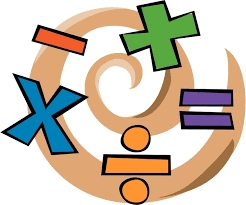We would be pleased if you find an error in the word problem, spelling mistakes, or inaccuracies and send it to us. Thank you!

Showing 1 comment:Math student
km=45?.Tips to related online calculators
Need help calculate sum, simplify or multiply fractions? Try our fraction calculator.

## Next similar math problems:

• Here isHere is a data set (n=117) that has been sorted. 10.4 12.2 14.3 15.3 17.1 17.8 18 18.6 19.1 19.9 19.9 20.3 20.6 20.7 20.7 21.2 21.3 22 22.1 22.3 22.8 23 23 23.1 23.5 24.1 24.1 24.4 24.5 24.8 24.9 25.4 25.4 25.5 25.7 25.9 26 26.1 26.2 26.7 26.8 27.5 27.6 2
• Expressions with variableThis is algebra. Let n represent an unknown number and write the following expressions: 1. 4 times the sum of 7 and the number x 2. 4 times 7 plus the number x 3. 7 less than the product of 4 and the number x 4. 7 times the quantity 4 more than the number
• Algebra problemThis is algebra. Let n represent an unknown number. 1. Eight more than the number n 2. Three times the number n 3. The product of the number n and eight 4. Three less than the number n 5. Three decreased by the number n
• NumbersDetermine the number of all positive integers less than 4183444 if each is divisible by 29, 7, 17. What is its sum?
• Chickens and rabbitsIn the yard were chickens and rabbits. Together they had 27 heads and 86 legs. How many chickens and how many rabbits were in the yard?
• Speed of Slovakian trainsRudolf decided to take the train from the station 'Ostratice' to 'Horné Ozorovce'. In the train timetables found train Os 5409 : km 0 Chynorany 15:17 5 Ostratice 15:23 15:23 8 Rybany 15:27 15:27 10 Dolné Naštice 15:31 15:31 14 Bánovce nad Bebravou 15:35 1
• DiophantusWe know little about this Greek mathematician from Alexandria, except that he lived around 3rd century A. D. Thanks to an admirer of his, who described his life through an algebraic riddle, we know at least something about his life. Diophantus's youth las
• Turtles 2A box turtle hibernates in the sand at 11 5/8. A spotted turtle hibernates at 11 16/25 feet. Which turtle is deeper?
• RWYCalculate the opposite direction of the runway 13. Runways are named by a number between 01 and 36, which is generally one tenth of the azimuth of the runway's heading in degrees: a runway numbered 09 points east (90°), runway 18 is south (180°), runway 2
• Christmas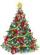Calculate how much of the school year (202 days long) take Christmas holidays 19 days long. Expressed as a decimal number and as a percentage.
• Reminder and quotientThere are given numbers A = 135, B = 315. Find the smallest natural number R greater than 1 so that the proportions R:A, R:B are with the remainder 1.On the meadow grazing horses, cows and sheep, together less than 200. If cows were 45 times more, horses 60 times more and sheep 35 times more than there are now, their numbers would equall. How many horses, cows and sheep are on the meadow together?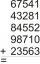Fill letters instead of digits so the indicated sum (equal letters represent equal digits). What number is hidden under the letter J? A A H A H O A H O J -------------------------- 4 3 2 1Calculate fixed annual personnel costs of operating monorail line 118 km long if every 5 km is station, which serve three people - one dispatcher and two switchman in 4-shift operation. Consider the average salary of the employee 885 €.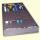Letter game Logik is a two player game, which has the following rules: 1. The first player thinks five-letter word in which no letter is not repeated. 2. The second player writes a five-letter word. 3. The first player answers two numbers - the first numbIf the quotient of 8/13 and 2 is subtracted from the product of 1 3/4 and 8/21, what is the difference?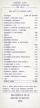Estimate the cost of the state based on following reason. State price is expressed as a percentage respectively share in the redistribution of national GDP. Employee creates monthly value € 868 (super-gross wage).Then tax him 35.2% employer contributions